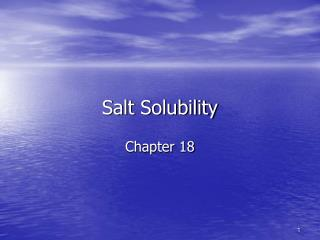DownloadDownload PresentationSalt Solubility

# Salt Solubility

Download Presentation## Salt Solubility

- - - - - - - - - - - - - - - - - - - - - - - - - - - E N D - - - - - - - - - - - - - - - - - - - - - - - - - - -
##### Presentation Transcript

1. Salt Solubility Chapter 18

2. Solubility product constant • Ksp • Unitless • CaF2(s) Ca2+(aq) + 2F-(aq) • Ksp = [Ca2+][F-]2 • [Ca2+] = molar solubility (in M) • Get Ksp values from Appendix J, pages A-24-25

3. We have problems • Ksp for BaSO4 = 1.1 x 10-10 @ 25°C • Calculate this salt’s solubility in water in g/L.

4. Solution

5. Problem • If [Ca2+] = 2.4 x 10-4 M, what is the Ksp value of CaF2?

6. Solution

7. Solubility and the Common Ion Effect • Problem: How much AgCl (g/L) would dissolve in water given Ksp= 1.8 x 10-10?

8. Solubility and the Common Ion Effect • If solid AgCl is placed in 1.00L of 0.55M NaCl, what mass of AgCl (g/L) would dissolve? • Before solving this, would you expect it to be lesser or greater than in pure water? • Think back to Le Châtelier’s Principle and the pH of buffers

9. Basic anions and salt solubility • Do we recall what a conjugate acid/conjugate base is? • If salt has conjugate base of weak acid, then salt more soluble than value given by Ksp • Why? PbS(s) Pb2+(aq) + S2-(aq) S2-(aq) + H2O(l) HS-(aq) + OH-(aq); Where net = Ksp  Kb (>Ksp)

10. Effect of pH on solubility • Mg(OH)2 (s) Mg2+(aq) + 2OH-(aq) • If one adds base, OH-, which way will the equilibrium shift? • Will more solid form or dissolve? • If one adds acid, H3O+, which way will the equilibrium shift? • Will more solid form or dissolve?

11. Effect of pH on solubility • Mg3(PO4)2 (s) 3Mg2+(aq) + 2PO4-3(aq) • If one adds base, OH-, which way will the equilibrium shift? • Will more solid form or dissolve? • If one adds acid, H3O+, which way will the equilibrium shift? • Will more solid form or dissolve?

12. Effect of pH on solubility • MgCl2 (s) Mg2+(aq) + 2Cl-(aq) • If one adds base, OH-, which way will the equilibrium shift? • Will more solid form or dissolve? • If one adds acid, H3O+, which way will the equilibrium shift? • Will more solid form or dissolve?

13. So what can we say about the conjugate base and solubility?

14. Q tells us: Whether it’s at equilibrium If not, which way it’ll shift CaF2(s) Ca2+(aq)+ 2F-(aq) Ksp = [Ca2+][F-]2 Q = [Ca2+][F-]2 If Q = Ksp, then @ eq.& soln is saturated If Q < Ksp, then will shift to right (dissolve more) & soln is unsaturated If Q > Ksp, then will shift to left (not dissolve anymore, ppt out) & soln is supersaturated (will ppt out) The reaction quotient, Q

15. Check out the heating pads & video • http://genchem.chem.wisc.edu/demonstrations/Gen_Chem_Pages/11solutionspage/crystallization_from_super.htm

16. Problem • AgCl placed in water. After a certain amount of time, concentration= 1.2 x 10-5 M. • Has the system reached eq.? • If not, will more solid dissolve? • How much more? • (Ksp = 1.8 x 10-10)

17. Selective precipitation • Will a precipitate form, given the ion concentrations? • What concentrations of ions are needed to bring about precipitation? • Basically, compare Q to Ksp

18. Problem • If [Mg2+] = 1.5x10-6 M, and enough hydroxide ions are added to make the solution 1.0 x 10-4 M of hydroxide ions, will Mg(OH)2 precipitate? • If not, will it occur if the concentration of hydroxide ions is increased to 1.0 x 10-2 M? • Ksp = 5.6 x 10-12

19. Complex ion equilibria • Complex ion = a central metal ion (e- acceptor = Lewis acid) bound to 1 or more ligands • Ligand = neutral molecule or ion that acts as Lewis base (e- donor) with the central metal ion • Kf values on page A-26, Appendix K

20. Example • What is Knet for the reaction below?

21. Problem • What is the net rxn and value of Knet for dissolving AgBr in a solution containing S2O32-? • Ksp = 5.4 x 10-13 • Kf = 2.0 x 1013